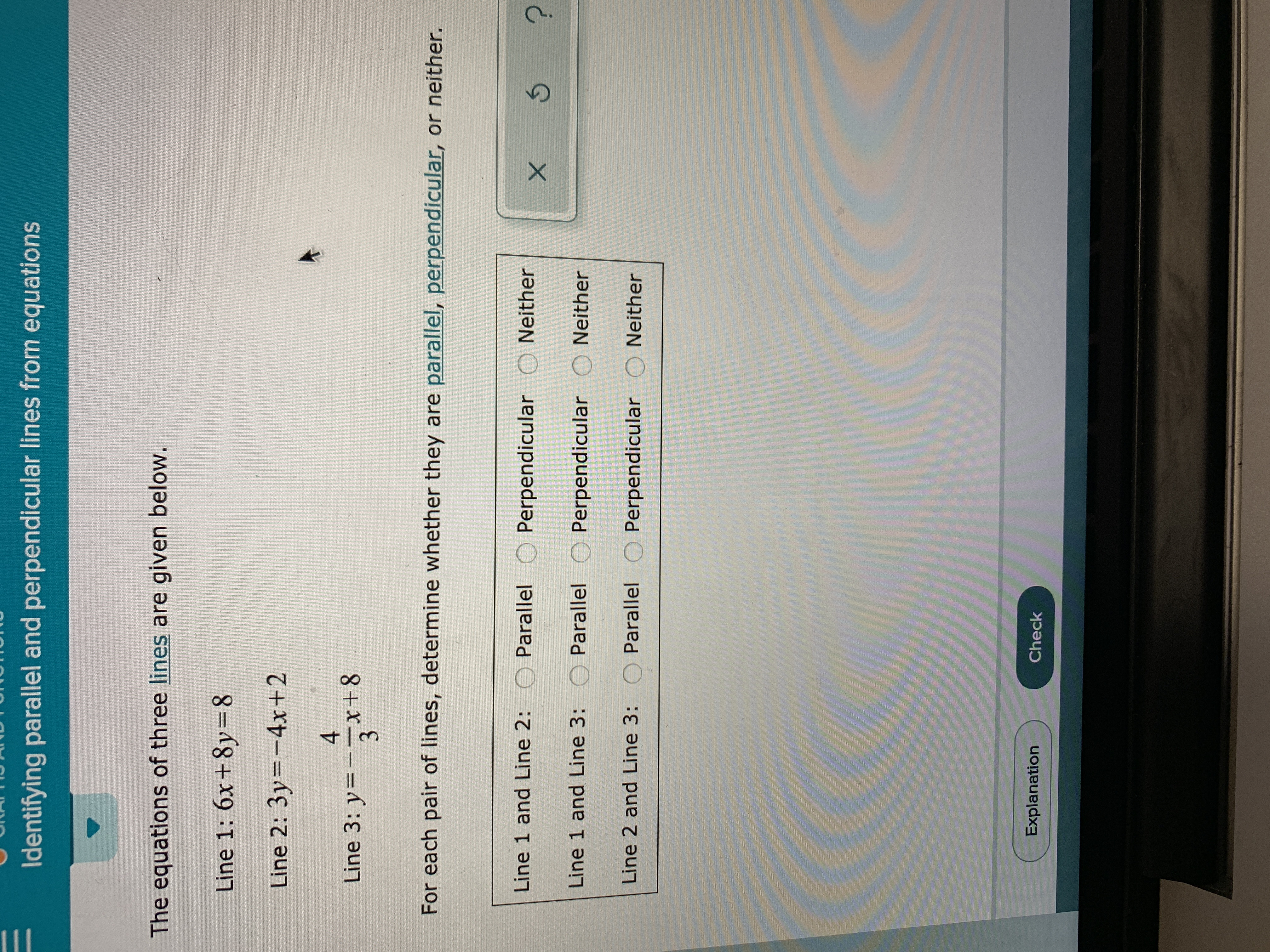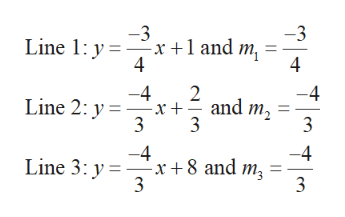# Identifying parallel and perpendicular lines from equationsThe equations of three lines are given below.Line 1: 6x+ 8y=8Line 2: 3y-4x+24Line 3: y=x+8For each pair of lines, determine whether they are parallel, perpendicular, or neither.Line 1 and Line 2: O ParallelPerpendicularNeitherLine 1 and Line 3: OParallel Perpendicular ONeitherLine 2 and Line 3:OParallel OPerpendicular O NeitherExplanationCheckX

Question
193 viewshelp_outlineImage TranscriptioncloseIdentifying parallel and perpendicular lines from equations The equations of three lines are given below. Line 1: 6x+ 8y=8 Line 2: 3y-4x+2 4 Line 3: y= x+8 For each pair of lines, determine whether they are parallel, perpendicular, or neither. Line 1 and Line 2: O Parallel Perpendicular Neither Line 1 and Line 3: O Parallel Perpendicular ONeither Line 2 and Line 3: O Parallel OPerpendicular O Neither Explanation Check X fullscreen
check_circle

Step 1

Calculation:

Rewrite each given equation of lines in the slope intercept form...help_outlineImage Transcriptionclose-3 3 Line 1: y xand m 4 -4 Line 2: y 3 2 -4 and m 3 3 -4 -x8 and m 3 -4 Line 3: y 3 4 fullscreen

### Want to see the full answer?

See Solution

#### Want to see this answer and more?

Solutions are written by subject experts who are available 24/7. Questions are typically answered within 1 hour.*

See Solution
*Response times may vary by subject and question.
Tagged in